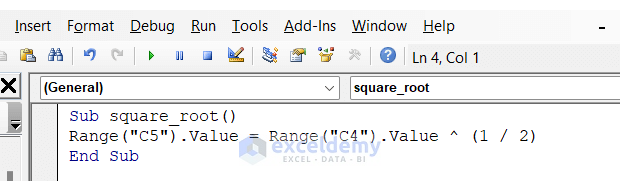# Difference Between Subroutine and Function in Excel VBA

Get FREE Advanced Excel Exercises with Solutions!

There are lots of similarities between a custom Function/User-Defined Function procedure and the Sub-procedure/Subroutine in Excel. However, Function procedures have some important differences from Subroutine procedures. In this article, we will see the difference between subroutine and function in Excel VBA.

## Introduction to Subroutine and Function in Excel VBA

The most important difference is that a function returns a value (a number or a text string). The value of the function procedure is stored in a variable; a variable whose name is the same as the function’s name. The subroutine performs some set of tasks and does not return a value like functions.

### 1. Excel VBA User-Defined Function

See the following example. AddTwoNumber is the function name. This function will return the sum of two numbers passed as arguments (arg1 and arg2). The sum is stored in a variable named AddTwoNumber same as the function name.To create a custom function, follow these steps:

• Firstly, activate the VBA Editor by pressing Alt+F11.
• Secondly, select the workbook in the Project window.
• Thirdly, choose Insert and then Module to insert a VBA You can also use an existing code module. The code module must be a standard VBA module.• Then copy and paste the below code for the function. The function name must be unique for that workbook. Enter a list of the arguments (if any) in parentheses. If the function doesn’t use an argument, the VBA Editor adds a set of empty parentheses.
``````Function AddTwoNumber(arg1, arg2)
'Returns the sum of two numbers you supply as arguments
End Function``````
• Furthermore, this part is important. Insert the VBA code that performs your intended objective. The value that you want to return from this function will be stored in a variable; a variable whose name is the same as the function’s name.
• Finally, end the function with an End Function.Read More: How to Use VBA User Defined Function

### 2. Excel VBA Subroutine

In the following example, you will see how the subroutine in Excel VBA works. Here the Sub starts the body of the subroutine. The subroutine name is square_root. In the body of the subroutine, we perform a task in cell A2. The task is performing the square root in cell A2. It means, that if the cell contains any number, the Excel VBA will perform the square root of that cell. The End Sub ends the body of the subroutine.

To create a subroutine, follow these steps:

• First, activate the VBA Editor (press Alt+F11).
• Second, select the workbook in the Project window.
• Third, choose Insert and then Module to insert a VBA module. You can also use an existing code module. The code module must be a standard VBA module.• Next, enter the keyword SUB followed by the Subroutine’s name.
• In addition, insert the VBA code that you want to perform.
``````Sub square_root()
Range("C5").Value = Range("C4").Value ^ (1 / 2)
End Sub``````
• Finally, the Subroutine with an End Sub.
``

## Key Differences Between Subroutine & Function in Excel VBA

After performing the subroutine and functions separately we can conclude the differences in the table below.

Functions Subroutines
1) Returns a value. 1) Performs a set of tasks but doesn’t return a value.
2) Functions are called by using a variable. 2) Can be recalled from anywhere within the program in multiple types after the declaration.
3) Can be used as formulas in the spreadsheets. 3) Cannot be used directly in the spreadsheets as formulas.
4) We can use functions as formulas in the spreadsheets. We can perform it several times after running the code. 4) To find the result of the Excel VBA subroutine we have to insert a value in the desired cell first.
5) Syntax:

Function Function_Name()

//Set of codes

End Function

5) Syntax:

Sub Sub_Name ()

//Set of codes

End Sub

Read More: VBA Sub Vs Function in Excel

## Things to Remember

• The Developer tab must be enabled before using these methods.
• We can find Subroutine in Macros in the Developer tab while user-defined functions in the Function tab using custom search.

## Conclusion

If you’re still having trouble with any of these instructions or having discrepancies, let us know in the comments. Our team is ready to answer all of your questions.

## What is ExcelDemy?

ExcelDemy Learn Excel & Excel Solutions Center provides free Excel tutorials, free support , online Excel training and Excel consultancy services for Excel professionals and businesses. Feel free to contact us with your Excel problems.Kawser Ahmed

Hello! Welcome to my Excel blog! It took me some time to be a fan of Excel. But now I am a die-hard fan of MS Excel. I learn new ways of doing things with Excel and share them here. Not only a how-to guide on Excel, but you will get also topics on Finance, Statistics, Data Analysis, and BI. Stay tuned! You can check out my courses at Udemy: udemy.com/user/exceldemy/

1 CommentAdvanced Excel Exercises with Solutions PDF